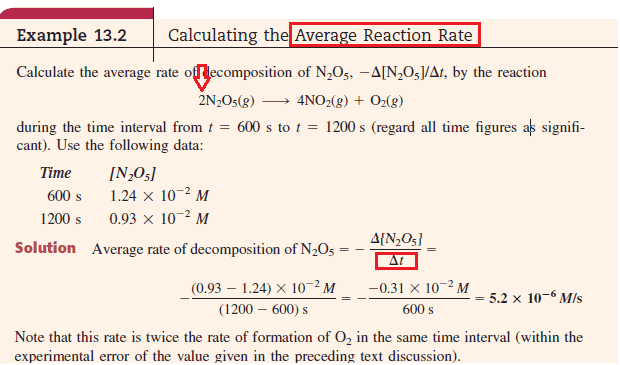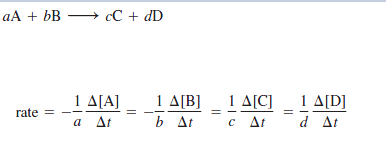# Calculation of the average rate of reaction!

Hi,

Im stuck: I found this example in which they calculate the rate of reaction: the title is "calculating the average reaction rate"

Now how the hell is this average reaction rate if they didnt use stoichiometric coefficients!? (in this case it should be 2.Hi,
Now how the hell is this average reaction rate if they didnt use stoichiometric coefficients!? (in this case it should be 2.

Why and how are the stoichiometric coefficients to be used?

because the average rate always is calculated using this formula:True that is the rate of the reaction.

The question asks, instead, for rate of decomposition of the reactant. Which is simply the rate of change of its concentration with time.

True that is the rate of the reaction.

The question asks, instead, for rate of decomposition of the reactant. Which is simply the rate of change of its concentration with time.

Thanks, So u mean that it is not the rate of the reaction that is calculated in this example?

Thanks, So u mean that it is not the rate of the reaction that is calculated in this example?

Yes, that is correct. They have merely found the rate of change of concentration of the reactant (which in this case is because of decomposition. Note that if they had asked the rate of formation of one of the products, it would simply again be rate of change in its concentration with respect to time)

And what if they would ask "the rate of disappearance"? Do u have any idea what that might mean? Thanks!

Disappearance or appearance would simply mean change in concentration (in the first case, the conc. is decreasing and in the second it is increasing). So you just have to find rate of change of concentration.

Note: Some textbooks use the convention that rate of disappearance is $-\frac{dC}{dt}$
where C is the concentration. For appearance the expression is similar but with the absence of the minus sign.